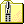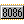# MatLab 1.x (DOS)

MatLab is a high speed, interactive programming utility for manipulating, calculating, and plotting complicated mathematical equations. It is considered easy to use for those familiar with typical algebra equations.

It competed against Mathematica.

Early versions were copy protected.

Product type
Application Mathematics
Vendor
MathWorks
Release date
1986
Minimum CPU
8088
User interface
Text
Platform
DOSMatLab 1.0 for DOS 1.0 English137.27KB 1